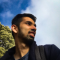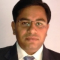## Basic approaches for Data generalization (DWDM)Updated on 10-Jan-2023 17:14:04
Data generalization, also known as data summarization or data compression, is the process of reducing the complexity of large datasets by identifying and representing patterns in the data in a more simplified form. This is typically done in order to make the data more manageable and easier to analyze and interpret. Introduction to Data Generalization Data generalization is a crucial step in the data analysis process, as it allows us to make sense of large and complex datasets by identifying patterns and trends that may not be immediately apparent. By simplifying the data, we can more easily identify relationships, classify ... Read More

## How to implement linear classification with Python Scikit-learn?Updated on 04-Oct-2022 08:40:49
Linear classification is one of the simplest machine learning problems. To implement linear classification, we will be using sklearn’s SGD (Stochastic Gradient Descent) classifier to predict the Iris flower species. Steps You can follow the below given steps to implement linear classification with Python Scikit-learn − Step 1 − First import the necessary packages scikit-learn, NumPy, and matplotlib Step 2 − Load the dataset and build a training and testing dataset out of it. Step 3 − Plot the training instances using matplotlib. Although this step is optional, it is good practice to plot the instances for more clarity. Step 4 − Create ... Read More

## How to transform Scikit-learn IRIS dataset to 2-feature dataset in Python?Updated on 04-Oct-2022 08:38:18
Iris, a multivariate flower dataset, is one of the most useful Pyhton scikit-learn datasets. It has 3 classes of 50 instances each and contains the measurements of the sepal and petal parts of three Iris species namely Iris setosa, Iris virginica, and Iris versicolor. Along with that Iris dataset contains 50 instances from each of these three species and consists of four features namely sepal_length (cm), sepal_width (cm), petal_length (cm), petal_width (cm). We can use Principal Component Analysis (PCA) to transform IRIS dataset into a new feature space with 2 features. Steps We can follow the below given steps to ... Read More

## How to transform Sklearn DIGITS dataset to 2 and 3-feature dataset in Python?Updated on 04-Oct-2022 08:35:06
Sklearn DIGITS dataset has 64 features as each image of the digit is of size 8 by 8 pixels. We can use Principal Component Analysis (PCA) to transform Scikit-learn DIGITS dataset into new feature space with 2 features. Transforming 64 features dataset to 2-feature dataset will be a big reduction in the size of data and we’ll lose some useful information. It will also impact the classification accuracy of ML model. Steps to Transform DIGITS Dataset to 2-feature Dataset We can follow the below given steps to transform DIGITS dataset to 2-feature dataset using PCA − First, import the ... Read More

## How to perform dimensionality reduction using Python Scikit-learn?Updated on 04-Oct-2022 08:32:09
Dimensionality reduction, an unsupervised machine learning method is used to reduce the number of feature variables for each data sample selecting set of principal features. Principal Component Analysis (PCA) is one of the popular algorithms for dimensionality reduction available in Sklearn. In this tutorial, we perform dimensionality reduction using principal component analysis and incremental principal component analysis using Python Scikit-learn (Sklearn). Using Principal Component Analysis (PCA) PCA is a statistical method that linearly project the data into new feature space by analyzing the features of original dataset. The main concept behind PCA is to select the “principal” characteristics of the ... Read More

## How to implement Random Projection using Python Scikit-learn?Updated on 04-Oct-2022 08:29:24
Random projection is a dimensionality reduction and data visualization method to simplify the complexity of highly dimensional data. It is basically applied to the data where other dimensionality reduction techniques such as Principal Component Analysis (PCA) can not do the justice to data. Python Scikit-learn provides a module named sklearn.random_projection that implements a computationally efficient way to reduce the data dimensionality. It implements the following two types of an unstructured random matrix − Gaussian Random Matrix Sparse Random Matrix Implementing Gaussian Random Projection For implementing Gaussian random matrix, random_projection module uses GaussianRandomProjection() function which reduces the dimensionality by ... Read More

## How to build Naive Bayes classifiers using Python Scikit-learn?Updated on 04-Oct-2022 08:25:42
Naïve Bayes classification, based on the Bayes theorem of probability, is the process of predicting the category from unknown data sets. Scikit-learn has three Naïve Bayes models namely, Gaussian Naïve Bayes Bernoulli Naïve Bayes Multinomial Naïve Bayes In this tutorial, we will learn Gaussian Naïve Bayes and Bernoulli Naïve Bayes classifiers using Python Scikit-learn (Sklearn). Gaussian Naïve Bayes Classifier Gaussian naïve bayes classifier is based on a continuous distribution characterized by mean and variance. With the help of an example, let’s see how we can use the Scikit-Learn Python ML library to build a Gaussian Naïve Bayes classifier. ... Read More

## How to create a random forest classifier using Python Scikit-learn?Updated on 04-Oct-2022 08:22:46
Random forest is a supervised machine learning algorithm that is used for classification, regression, and other tasks by creating decision trees on data samples. After creating the decision trees, a random forest classifier collects the prediction from each of them and selects the best solution by means of voting. One of the best advantages of a random forest classifier is that it reduces overfitting by averaging the result. That is the reason we get better results as compared to a single decision tree. Steps to Create Random Forest Classifier We can follow the below steps to create a random forest ... Read More

## How to get dictionary-like objects from dataset using Python Scikit-learn?Updated on 04-Oct-2022 08:19:08
With the help of the Scikit-learn python library, we can get the dictionary-like objects of a dataset. Some of the interesting attributes of dictionary-like objects are as follows − data − It represents the data to learn. target − It represents the regression target. DESCR − The description of the dataset. target_names − It gives the target names on of the dataset. feature_names − It gives the feature names from the dataset. Example 1 In the example below we use the California Housing dataset to get its dictionary-like objects. # Import necessary libraries import sklearn import pandas as ... Read More

## How to binarize the data using Python Scikit-learn?Updated on 04-Oct-2022 08:16:38
Binarization is a preprocessing technique which is used when we need to convert the data into binary numbers i.e., when we need to binarize the data. The scikit-learn function named Sklearn.preprocessing.binarize() is used to binarize the data. This binarize function is having threshold parameter, the feature values below or equal this threshold value is replaced by 0 and value above it is replaced by 1. In this tutorial, we will learn to binarize data and sparse matrices using Scikit-learn (Sklearn) in Python. Example Let’s see an example in which we preprocess a numpy array into binary numbers − # Importing ... Read More